### 简单线性回归

0.前提介绍
为什么需要统计量？
统计量：描述数据特征
0.1 集中趋势衡量
0.1.1 均值 （平均数，平均值）（mean）
{6,2,9,1,2}
(6 + 2 + 9 + 1+2)/5 = 20/5 = 4
0.1.2 中位数 （median）
将数据中的各个数值按照大小顺序排列，居于中间位置的变量
0.1.2.1 给数据排序： 1，2，2，6，9
0.1.2.2 找出位置处于中间的变量：2
当n为奇数时，直接取中间的变量
当n为偶数时，取中间两个量的平均值
0.1.3 众数 （mode）
数据中出现次数最多的数
0.2
0.2.1 离散程序衡量
0.2.1.1 方差 （variance）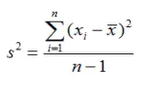{6,2,9,1,2}
(1) (6-4)^2 + (2-4)^2 + (9-4)^2 + (1-4)^2 + (2-4)^2
= 4 + 4 + 25 + 9 + 4
= 46
(2) n – 1 = 5 -1 = 4
(3) 46 / 4 = 11.5
0.2.1.2 标准差 （standard deviation）s = sqrt(11.5) = 3.39
1.介绍 ： 回归(regression) Y 变量为连续数值型（continuous numerical variable）
如：房价 ，人数 ， 降雨量
分类(Classification):Y 变量为类别型 (categorical variable)
如：颜色类别 ， 电脑品牌，有无信誉
2.简单线性回归(Simple Linear Regression)
2.1很多做决定过程通常是根据两个或者多个变量之间的关系
2.2回归分析(regression analysis)用来建立方程模拟两个或者多个变量之间如何关联
2.3被预测的变量叫做：因变量(dependent variable),y,输出(output)
2.4被用来进行预测的变量叫做：自变量（independent variable）,x ,输入(input)
3.简单线性回归介绍
3.1简单线性回归包含一个自变量（x）和一个因变量（y）
3.2以上两个变量的关系用一条直线来模拟
3.3如果包含两个以上的自变量，则称作多元回归分析（multiple regression）
4.简单线性回归模型
4.1被用来描述因变量（y）和自变量（x）以及偏差（error）之间关系的方程叫做回归模型
4.2简单线性回归的模型是：
y = b0 + b1x + e
b0 、 b1为变量 ， e为偏差值，满足正态分布
5.简单线性回归方程
E(y) = B0 + B1x
这个方程对应的图像是一条直线 ，称作回归线
其中，B0是回归线的截距
B1是回归线的斜率， E(y)是在一个给定x值下y的期望值（均值）

6.正向线性关系：7.负向线性关系8.无关系9.估计线性回归方程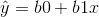这个方程叫做估计线性方程（estimated regression line）
其中，b0是估计线性方程的纵截距，b1是估计线性方程的斜率是在自变量x等于一个给定值的时候，y的估计值

10.线性回归分析流程：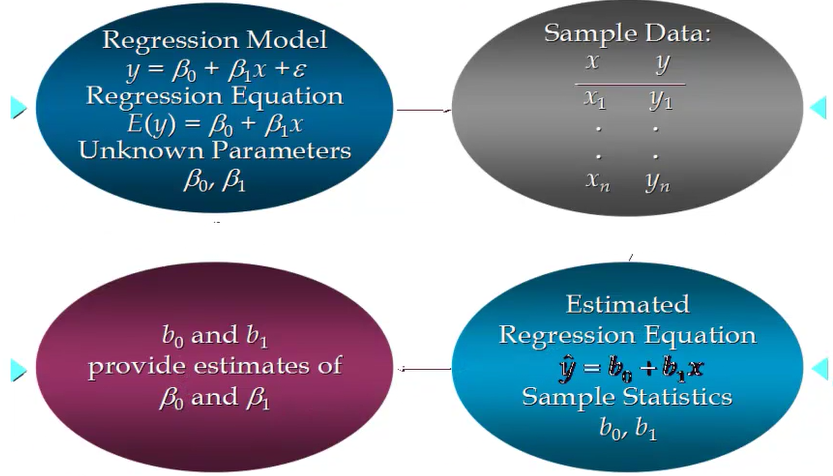11.关于偏差e的假定
11.1是一个随机的变量，均值为0
11.2e的方差（variance）对于所有的自变量x是一样的
11.3e的值是独立的
11.4e满足正态分布

12.简单线性回归模型举例：

 ads sold 1 14 3 24 2 18 1 17 3 27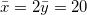1.1如何找到简单线性回归模型的最佳回归线？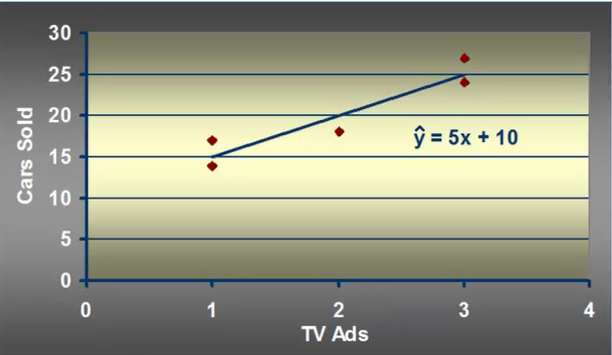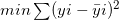目的是找到一条直线 ，使得sum of squares最小

1.先计算出x和y的平均值，即

2.将每组数据的x值和y值分别与对应的平均值相减，再将相减得到的值相乘、加总计算，作为b1的分子

3.计算每组数据的x值减去x的平均值，再将结果值平方，最后加总计算，作为b1的分母

4.将每2步比上第3步，得出变量值b1

5.最后将b1带入直接线回归方程 y = b0 + b1x ,算出b0结果，就可以得到方程式了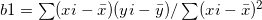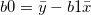= 6 + 4 + 0 + 3 + 7

= 20

= 1+1+0+1+1

= 4

b1 = 20/4 = 5

b0 = 20 – 5 * 2 = 20 – 10 = 10

Tags :